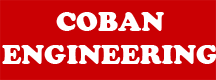Forces in Metal Cutting: Equations can be derived to relate the forces that cannot be measured to the forces that can be measured:

F = Fc x sin α + Ft x cos α
N = Fc x cos α - Ft x sin α
Fs = Fc x cos ф - Ft x sinф
Fn = Fc x sinф + Ft x cosф

Based on these calculated force, shear stress and coefficient of friction can be determined."F" (frictional force ) and "Fn" (Force normal to the Shear force; the resistance offered by the work-piece) can be used to define the coefficient of friction between the cutting tool and the chip. The friction force and its normal force can be added vectorially to form a resultant force, R. R is oriented at an angle, called the friction angle. The coefficient of friction and friction angle can be related and calculated as follows: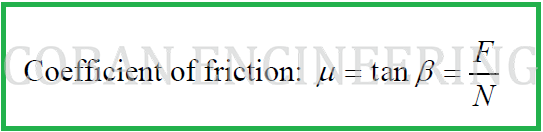For the forces acting on the chip to be in balance, the resultant forces, R, must be colinear, opposite in direction and equal in magnitude. If cutting force and thrust force are known, these four equations can be used to calculate estimates of shear force, friction force, and normal force to friction. Based on these force estimates (F, N, Fs ),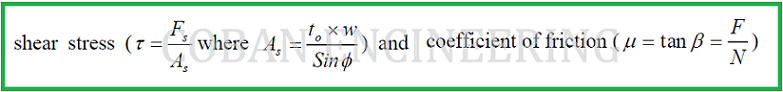can be determined.

Example: Suppose that cutting force and thrust force are measured during an orthogonal cutting operation with values Fc = 350 N and Ft =285 N. The width of the orthogonal cutting operation w = 0.125 cm, to = 0.020cm, ф=25.4° , α= 10°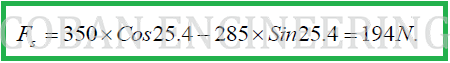The shear plane area is calculated as: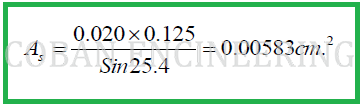Thus, the sear stress, which equals the shear strength of the work material, is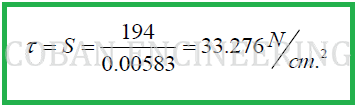If the shear strength (S) of the work material is known, cutting force (Fc) and thrust force (Ft) in an orthogonal cutting operation can be estimated:

Known:Calculate the cutting force and the thrust force: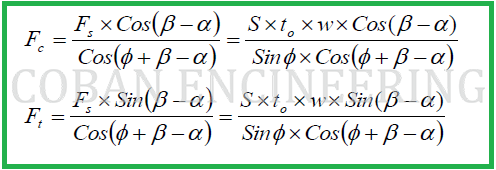Untitled 1 Footer

Home | Mechanical Engineering| Robot | Aerospace | Structural Engineering |Videos | Comment |